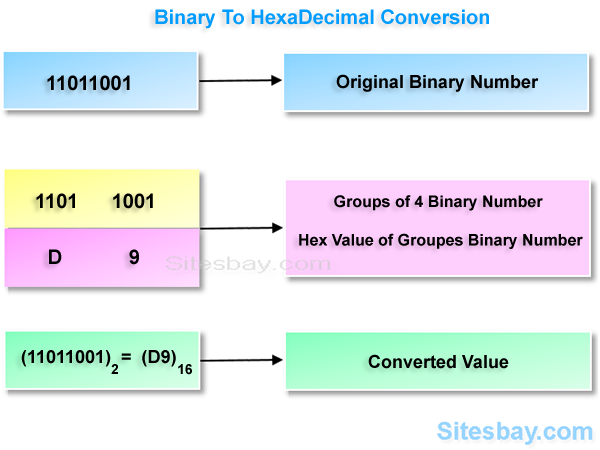# Convert Binary to Hexadecimal in C

## Convert Binary to Hexadecimal Program in C

In this types of program we takes a binary number as input and converts it into Hexadecimal number.

Binary NumberBinary number system is a base 2 number system. Binary number system uses only two symbols to represent all its values i.e. 0 and 1

HexaDecimal NumberHexadecimal number system is base 16 number system. Hexadecimal number system uses 16 symbols to represent all its values i.e. 1B

### Binary to Hexadecimal Covertion Method

• Take a binary number as input.
• Group all binary bits to 4 digits starting from right side.
• Write corresponding hexadecimal value of each grouped digit.000000
100011
200102
300113
401004
501015
601106
701117
810008
910019
101010A
111011B
121100C
131101D
141110E
151111F

## Convert Binary to Hexadecimal in C

```#include<stdio.h>
#include<conio.h>

void main()
{
long int binary_number, hexadecimal_number = 0, i = 1, remainder;
clrscr();
printf("Please Enter any Binary Number: ");
scanf("%ld", &binary_number);
while (binary_number != 0)
{
remainder = binary_number % 10;
i = i * 2;
binary_number = binary_number / 10;
}

getch();
}
```

## Output 1

```Please Enter any Binary Number: 10011
```

## Output 2

```Please Enter any Binary Number: 11011
```

WIFI CCTV Camera